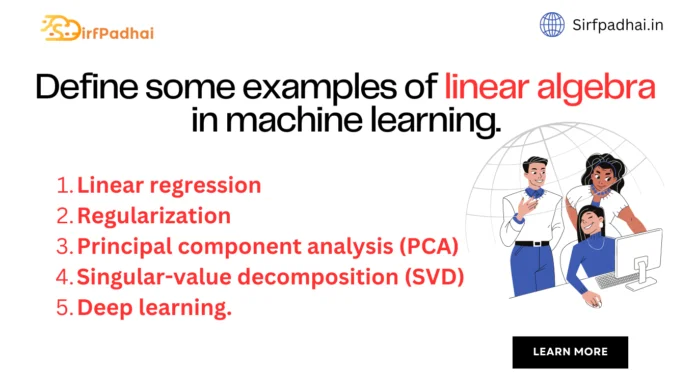# Define some examples of linear algebra in machine learning.## Define some examples of linear algebra in machine learning.

Some examples of linear algebra in machine learning are as follows-

1. Linear regression
2. Regularization
3. Principal component analysis (PCA)
4. Singular-value decomposition (SVD)
5. Deep learning.

### (i) Linear Regression –

It is an old method from statistics for describing the relationships between variables. It is often used in machine learning for predicting numerical values in simpler regression problems. There are many ways to describe and solve the linear regression problem, i.e. finding a set of coefficients that when multiplied by each of the input variables and added together results in the best prediction of the output variable. If you have used a machine learning tool or library, the most common way of solving linear regression is via a least squares optimization that is solved using matrix factorization methods from linear regression, such as an LU decomposition or a singular-value decomposition or SVD. Even the common way of summarizing the linear regression equation uses linear algebra notation

x= B.a

where x is the output variable B is the dataset and a are the model coefficients.

### (ii) Regularization –

In applied machine learning, we often seek the simplest possible models that achieve the best skill on our problem. Simpler models are often better at generalizing from specific examples to unseen data. In many methods that involve coefficients, such as regression methods and artificial neural networks, simpler models are often characterized by models that have smaller coefficient values. A technique that is often used to encourage a model to minimize the size of coefficients while it is being fit on data is called regularization. Common implementations include the L² and L¹ forms of regularization. Both of these forms of regularization are in fact a measure of the magnitude or length of the coefficients as a vector and are methods lifted directly from linear algebra called the vector norm.

### (iii) Principal Component Analysis (PCA) –

Often a dataset has many columns, perhaps tens, hundreds, thousands, or more. Modeling data with many features is challenging, and models built from data that include irrelevant features are often less skillful than models trained from the most relevant data. It is hard to know which features of the data are relevant and which are not. Methods for automatically reducing the number of columns of a dataset are called dimensionality reduction, and perhaps the most popular method is called the principal component analysis or PCA for short. This method is used in machine learning to create projections of high-dimensional data for both visualization and for training models. The core of the PCA method is a matrix factorization method from linear algebra. The eigen decomposition can be used and more robust implementations may use the singular-value decomposition or SVD.

### (iv) Singular –

value Decomposition (SVD) Another popular dimensionality reduction method is the singular-value decomposition method or SVD for short. As mentioned and as the name of the method suggests, it is a matrix factorization method from the field of linear algebra. It has wide use in linear algebra and can be used directly in applications such as feature selection, visualization, noise reduction, and more.

### (v) Deep Learning –

Artificial neural networks are nonlinear machine learning algorithms that are inspired by elements of the information processing in the brain and have proven effective at a range of problems not least predictive modeling. Deep learning is the recently resurged use of artificial neural networks with newer methods and faster hardware that allow for the development and training of larger and deeper (more layers) networks on very large datasets. Deep learning methods routinely achieve state-of-the-art results on a range of challenging problems such as machine translation, photo captioning, speech recognition, and much more At their core, the execution of neural networks involves linear algebra data structures multiplied and added together. Scaled up to multiple dimensions, deep learning methods work with vectors, matrices, and even tensors of inputs and coefficients, where a tensor is a matrix with more than two dimensions. Linear algebra is central to the description of deep learning methods via matrix notation to the implementation of deep learning methods such as Google’s TensorFlow Python library that has the word “tensor” in its name.

### Write short notes on statistics and linear algebra for ML.

Linear algebra is a valuable tool in other branches of mathematics, especially statistics.

The impact of linear algebra is important to consider, given the foundational relationship both fields have with the field of applied machine learning. Some points of linear algebra on statistics and statistical methods are as follows-

(i) Use of vector and matrix notation, especially with multivariate statistics.

(ii) Solutions to least squares and weighted least squares, such as for linear regression.

(iii) Estimates of mean and variance of data matrices.

(iv) The covariance matrix that plays a key role in multinomial Gaussian distributions.

(v) Principal component analysis for data reduction that draws many of these elements together.

As we can see, modern statistics and data analysis, at least as far as the interests of a machine learning practitioner are concerned, depend on the understanding and tools of linear algebra.